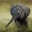Related Tags

c++

# How to rearrange a linked list in zig-zag orderChayshta Singh

### Overview

The following article will explain how to rearrange a linked list, so its elements are in zig-zag order.

If [a < c < d > b] is an input list, then one zig-zag order is [a < d > b < c].

### Algorithm

In this solution, we don’t consider the case where there are duplicate elements in the linked list.

We compare the linked list’s first three elements (zero, one, and two). If they are in ascending order (zero < one < two) or descending order (zero > one > two), we swap nodes one and two to obtain the zig-zag order. This results in (zero < two > one) and (zero > two < one ) respectively for ascending and descending order. Then we shift our current position by one step and consider the next three elements. This algorithm stops when we reach the end of the linked list, and the third element is NULL.

Consider the following test case where alternating colors represent zig-zag order.

1 of 7

### Example

The following code will demonstrate how to rearrange a link list in zig-zag order:

Node.h
#include <iostream>
#include "Node.h"
using namespace std;
/*
rearranges a linked list such that its elements are in a zig zag fashion.
*/
//zig-zag order atleast 3 elements
return;
//stops when less than 3 elements
while(zero->next != nullptr && zero->next->next != nullptr){
Node *one = zero->next;
Node *two = zero->next->next;

bool isNotZigZag = (zero->data < one->data) && (one->data < two->data);   //ascending order: zero < one < two
isNotZigZag = isNotZigZag || ( (zero->data > one->data) && (one->data > two->data));    //descending order: zero > one > two

//swaps two and one to break ascending/descending order into zig-zag order.
if(isNotZigZag){
one->next = two->next;
two->next = one;
zero->next = two;
}
zero = zero->next ;
}
}

// list values
int valuesList[] = {1,3,7,6,5,1};
int lengthList = 6;
Code

### Time complexity analysis

O(n) is the time complexity for the algorithm above, where n is the length of the linked list.

### Explanation

• Line 14: We loop to find three consecutive nodes of the list and stop when we reach the end of the link list.

• Lines 18 and 19: We find whether the current three nodes (zero, one, and two) are in ascending or descending order.

• Lines 22–26: We swap one and two to transform ascending/descending order into zig-zag.

RELATED TAGS

c++

CONTRIBUTORChayshta Singh
RELATED COURSES

View all Courses

Keep Exploring

Learn in-demand tech skills in half the time